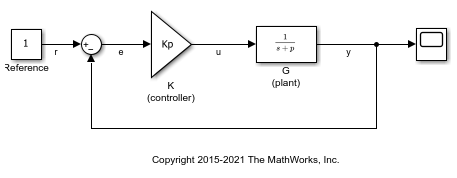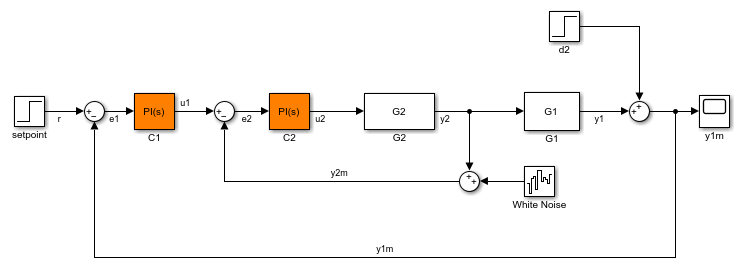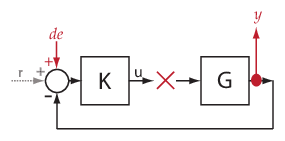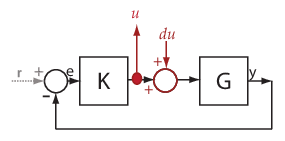Documentation

# getIOTransfer

Transfer function for specified I/O set using `slLinearizer` or `slTuner` interface

## Syntax

``linsys = getIOTransfer(s,in,out)``
``linsys = getIOTransfer(s,in,out,temp_opening)``
``linsys = getIOTransfer(s,ios)``
``linsys = getIOTransfer(___,mdl_index)``
``````[linsys,info] = getIOTransfer(___)``````

## Description

example

````linsys = getIOTransfer(s,in,out)` returns the transfer function for the specified inputs and outputs for the model associated with the `slLinearizer` or `slTuner` interface, `s`.The software enforces all the permanent openings specified for `s` when it calculates `linsys`. For information on how `getIOTransfer` treats `in` and `out`, see Transfer Functions. If you configured either `s.Parameters`, or `s.OperatingPoints`, or both, `getIOTransfer` performs multiple linearizations and returns an array of transfer functions.```

example

````linsys = getIOTransfer(s,in,out,temp_opening)` considers additional, temporary, openings at the point specified by `temp_opening`. Use an opening, for example, to obtain the transfer function of the controller in series with the plant, with the feedback loop open.```
````linsys = getIOTransfer(s,ios)` returns the transfer function for the inputs and outputs specified by `ios` for the model associated with `s`. Use the `linio` command to create `ios`. The software enforces the linearization I/O type of each signal specified in `ios` when it calculates `linsys`. The software also enforces all the permanent loop openings specified for `s`.```

example

````linsys = getIOTransfer(___,mdl_index)` returns a subset of the batch linearization results. `mdl_index` specifies the index of the linearizations of interest, in addition to any of the input arguments in previous syntaxes.Use this syntax for efficient linearization, when you want to obtain the transfer function for only a subset of the batch linearization results.```

example

``````[linsys,info] = getIOTransfer(___)``` returns additional linearization information.```

## Examples

collapse all

Obtain the closed-loop transfer function from the reference signal, `r`, to the plant output, `y`, for the `ex_scd_simple_fdbk` model.

Open the `ex_scd_simple_fdbk` model.

```mdl = 'ex_scd_simple_fdbk'; open_system(mdl); ```In this model:

``

Create an `slLinearizer` interface for the model.

```sllin = slLinearizer(mdl); ```

To obtain the closed-loop transfer function from the reference signal, `r`, to the plant output, `y`, add both points to `sllin`.

```addPoint(sllin,{'r','y'}); ```

Obtain the closed-loop transfer function from `r` to `y`.

```sys = getIOTransfer(sllin,'r','y'); tf(sys) ```
```ans = From input "r" to output "y": 3 ----- s + 8 Continuous-time transfer function. ```

The software adds a linearization input at `r`, `dr`, and a linearization output at `y`.`sys` is the transfer function from `dr` to `y`, which is equal to.

Obtain the plant model transfer function, `G`, for the `ex_scd_simple_fdbk` model.

Open the `ex_scd_simple_fdbk` model.

```mdl = 'ex_scd_simple_fdbk'; open_system(mdl); ```In this model:

``

Create an `slLinearizer` interface for the model.

```sllin = slLinearizer(mdl); ```

To obtain the plant model transfer function, use `u` as the input point and `y` as the output point. To eliminate the effects of feedback, you must break the loop. You can break the loop at `u`, `e`, or `y`. For this example, break the loop at `u`. Add these points to `sllin`.

```addPoint(sllin,{'u','y'}); ```

Obtain the plant model transfer function.

```sys = getIOTransfer(sllin,'u','y','u'); tf(sys) ```
```ans = From input "u" to output "y": 1 ----- s + 5 Continuous-time transfer function. ```

The second input argument specifies `u` as the input, while the fourth input argument specifies `u` as a temporary loop opening.`sys` is the transfer function from `du` to `y`, which is equal to.

Suppose you batch linearize the `scdcascade` model for multiple transfer functions. For most linearizations, you vary the proportional (`Kp2`) and integral gain (`Ki2`) of the `C2` controller in the 10% range. For this example, calculate the open-loop response transfer function for the inner loop, from `e2` to `y2`, for the maximum value of `Kp2` and `Ki2`.

Open the `scdcascade` model.

```mdl = 'scdcascade'; open_system(mdl); ```Create an `slLinearizer` interface for the model.

```sllin = slLinearizer(mdl); ```

Vary the proportional (`Kp2`) and integral gain (`Ki2`) of the `C2` controller in the 10% range.

```Kp2_range = linspace(0.9*Kp2,1.1*Kp2,3); Ki2_range = linspace(0.9*Ki2,1.1*Ki2,5); [Kp2_grid,Ki2_grid] = ndgrid(Kp2_range,Ki2_range); params(1).Name = 'Kp2'; params(1).Value = Kp2_grid; params(2).Name = 'Ki2'; params(2).Value = Ki2_grid; sllin.Parameters = params; ```

To calculate the open-loop transfer function for the inner loop, use `e2` and `y2` as analysis points. To eliminate the effects of the outer loop, break the loop at `e2`. Add `e2` and `y2` to `sllin` as analysis points.

```addPoint(sllin,{'e2','y2'}) ```

Determine the index for the maximum values of `Ki2` and `Kp2`.

```mdl_index = params(1).Value == max(Kp2_range) & params(2).Value == max(Ki2_range); ```

Obtain the open-loop transfer function from `e2` to `y2`.

```sys = getIOTransfer(sllin,'e2','y2','e2',mdl_index); ```

```mdl = 'scdcascade'; open_system(mdl) ```Create a linearization option set, and set the `StoreOffsets` option.

```opt = linearizeOptions('StoreOffsets',true); ```

Create `slLinearizer` interface.

```sllin = slLinearizer(mdl,opt); ```

Add analysis points to calculate the closed-loop transfer function.

```addPoint(sllin,{'r','y1m'}); ```

Calculate the input/output transfer function, and obtain the corresponding linearization offsets.

```[sys,info] = getIOTransfer(sllin,'r','y1m'); ```

View offsets.

```info.Offsets ```
```ans = struct with fields: x: [6x1 double] dx: [6x1 double] u: 1 y: 0 StateName: {6x1 cell} InputName: {'r'} OutputName: {'y1m'} Ts: 0 ```

## Input Arguments

collapse all

Interface to a Simulink model, specified as either an `slLinearizer` interface or an `slTuner` interface.

Input analysis point signal name, specified as:

• Character vector or string — Analysis point signal name.

To determine the signal name associated with an analysis point, type `s`. The software displays the contents of `s` in the MATLAB® command window, including the analysis point signal names, block names, and port numbers. Suppose that an analysis point does not have a signal name, but only a block name and port number. You can specify `in` as the block name. To use a point not in the list of analysis points for `s`, first add the point using `addPoint`.

You can specify `in` as a uniquely matching portion of the full signal name or block name. Suppose that the full signal name of an analysis point is `'LoadTorque'`. You can specify `in` as `'Torque'` as long as `'Torque'` is not a portion of the signal name for any other analysis point of `s`.

For example, `in = 'y1m'`.

• Cell array of character vectors or string array — Specifies multiple analysis point names. For example, ```in = {'y1m','y2m'}```.

Output analysis point signal name, specified as:

• Character vector or string — Analysis point signal name.

To determine the signal name associated with an analysis point, type `s`. The software displays the contents of `s` in the MATLAB command window, including the analysis point signal names, block names, and port numbers. Suppose that an analysis point does not have a signal name, but only a block name and port number. You can specify `out` as the block name. To use a point not in the list of analysis points for `s`, first add the point using `addPoint`.

You can specify `out` as a uniquely matching portion of the full signal name or block name. Suppose that the full signal name of an analysis point is `'LoadTorque'`. You can specify `out` as `'Torque'` as long as `'Torque'` is not a portion of the signal name for any other analysis point of `s`.

For example, `out = 'y1m'`.

• Cell array of character vectors or string array — Specifies multiple analysis point names. For example, ```out = {'y1m','y2m'}```.

Temporary opening signal name, specified as:

• Character vector or string — Analysis point signal name.

`temp_opening` must specify an analysis point that is in the list of analysis points for `s`. To determine the signal name associated with an analysis point, type `s`. The software displays the contents of `s` in the MATLAB command window, including the analysis point signal names, block names, and port numbers. Suppose that an analysis point does not have a signal name, but only a block name and port number. You can specify `temp_opening` as the block name. To use a point not in the list of analysis points for `s`, first add the point using `addPoint`.

You can specify `temp_opening` as a uniquely matching portion of the full signal name or block name. Suppose that the full signal name of an analysis point is `'LoadTorque'`. You can specify `temp_opening` as `'Torque'` as long as `'Torque'` is not a portion of the signal name for any other analysis point of `s`.

For example, `temp_opening = 'y1m'`.

• Cell array of character vectors or string array — Specifies multiple analysis point names. For example, ```temp_opening = {'y1m','y2m'}```.

Linearization I/Os, created using `linio`, specified as a linearization I/O object.

`ios` must specify signals that are in the list of analysis points for `s`. To view the list of analysis points, type `s`. To use a point that is not in the list of analysis points for `s`, you must first add the point to the list using `addPoint`.

For example:

```ios(1) = linio('scdcascade/setpoint',1,'input'); ios(2) = linio('scdcascade/Sum',1,'output'); ```

Here, `ios(1)` specifies an input, and `ios(2)` specifies an output.

Index for linearizations of interest, specified as:

• Array of logical values — Logical array index of linearizations of interest. Suppose that you vary two parameters, `par1` and `par2`, and want to extract the linearization for the combination of ```par1 > 0.5``` and `par2 <= 5`. Use:

```params = s.Parameters; mdl_index = params(1).Value>0.5 & params(2).Value <= 5;```

The expression `params(1).Value>0.5 & params(2).Value<5` uses logical indexing and returns a logical array. This logical array is the same size as `params(1).Value` and `params(2).Value`. Each entry contains the logical evaluation of the expression for corresponding entries in `params(1).Value` and `params(2).Value`.

• Vector of positive integers — Linear index of linearizations of interest. Suppose that you vary two parameters, `par1` and `par2`, and want to extract the linearization for the combination of ```par1 > 0.5``` and `par2 <= 5`. Use:

```params = s.Parameters; mdl_index = find(params(1).Value>0.5 & params(2).Value <= 5);```

The expression `params(1).Value>0.5 & params(2).Value<5` returns a logical array. `find` returns the linear index of every true entry in the logical array

## Output Arguments

collapse all

Transfer function for specified I/Os, returned as described in the following:

• If you did not configure `s.Parameters` and `s.OperatingPoints`, the software calculates `linsys` using the default model parameter values. The software uses the model initial conditions as the linearization operating point. `linsys` is returned as a state-space model.

• If you configured `s.Parameters` only, the software computes a linearization for each parameter grid point. `linsys` is returned as a state-space model array of the same size as the parameter grid.

• If you configured `s.OperatingPoints` only, the software computes a linearization for each specified operating point. `linsys` is returned as a state-space model array of the same size as `s.OperatingPoints`.

• If you configured `s.Parameters` and specified `s.OperatingPoints` as a single operating point, the software computes a linearization for each parameter grid point. The software uses the specified operating point as the linearization operating point. `linsys` is returned as a state-space model array of the same size as the parameter grid.

• If you configured `s.Parameters` and specified `s.OperatingPoints` as multiple operating point objects, the software computes a linearization for each parameter grid point. The software requires that `s.OperatingPoints` is the same size as the parameter grid specified by `s.Parameters`. The software computes each linearization using corresponding operating points and parameter grid points. `linsys` is returned as a state-space model array of the same size as the parameter grid.

• If you configured `s.Parameters` and specified `s.OperatingPoints` as multiple simulation snapshot times, the software simulates and linearizes the model for each snapshot time and parameter grid point combination. Suppose that you specify a parameter grid of size `p` and `N` snapshot times. `linsys` is returned as a state-space model array of size `N`-by-`p`.

Linearization information, returned as a structure with the following fields:

Linearization offsets, returned as `[]` if `s.Options.StoreOffsets` is `false`. Otherwise, `Offsets` is returned as one of the following:

• If `linsys` is a single state-space model, then `Offsets` is a structure.

• If `linsys` is an array of state-space models, then `Offsets` is a structure array with the same dimensions as `linsys`.

Each offset structure has the following fields:

FieldDescription
`x`State offsets used for linearization, returned as a column vector of length nx, where nx is the number of states in `linsys`.
`y`Output offsets used for linearization, returned as a column vector of length ny, where ny is the number of outputs in `linsys`.
`u`Input offsets used for linearization, returned as a column vector of length nu, where nu is the number of inputs in `linsys`.
`dx`Derivative offsets for continuous time systems or updated state values for discrete-time systems, returned as a column vector of length nx.
`StateName`State names, returned as a cell array that contains nx elements that match the names in `linsys.StateName`.
`InputName`Input names, returned as a cell array that contains nu elements that match the names in `linsys.InputName`.
`OutputName`Output names, returned as a cell array that contains ny elements that match the names in `linsys.OutputName`.
`Ts`Sample time of the linearized system, returned as a scalar that matches the sample time in `linsys.Ts`. For continuous-time systems, `Ts` is `0`.

If `Offsets` is a structure array, you can configure an LPV System block using the offsets. To do so, first convert them to the required format using `getOffsetsForLPV`. For an example, see Approximating Nonlinear Behavior Using an Array of LTI Systems.

Linearization diagnostic information, returned as `[]` if `s.Options.StoreAdvisor` is `false`. Otherwise, `Advisor` is returned as one of the following:

`LinearizationAdvisor` objects store linearization diagnostic information for individual linearized blocks. For an example of troubleshooting linearization results using a `LinearizationAdvisor` object, see Troubleshoot Linearization Results at Command Line.

collapse all

### Transfer Functions

A transfer function is an LTI system response at a linearization output point to a linearization input. You perform linear analysis on transfer functions to understand the stability, time-domain characteristics, or frequency-domain characteristics of a system.

You can calculate multiple transfer functions for a given block diagram. Consider the `ex_scd_simple_fdbk` model:You can calculate the transfer function from the reference input signal to the plant output signal. The reference input (also referred to as setpoint), `r`, originates at the Reference block, and the plant output, `y`, originates at the G block. This transfer function is also called the overall closed-loop transfer function. To calculate this transfer function, the software adds a linearization input at `r`, `dr`, and a linearization output at `y`.The software calculates the overall closed-loop transfer function as the transfer function from `dr` to `y`, which is equal to (I+GK)-1GK.

Observe that the transfer function from `r` to `y` is equal to the transfer function from `dr` to `y`.

You can calculate the plant transfer function from the plant input, `u`, to the plant output, `y`. To isolate the plant dynamics from the effects of the feedback loop, introduce a loop break (or opening) at `y`, `e`, or, as shown, at `u`.The software breaks the loop and adds a linearization input, `du`, at `u`, and a linearization output at `y`. The plant transfer function is equal to the transfer function from `du` to `y`, which is G.

Similarly, to obtain the controller transfer function, calculate the transfer function from the controller input, `e`, to the controller output, `u`. Break the feedback loop at `y`, `e`, or `u`.

You can use `getIOTransfer` to obtain various open-loop and closed-loop transfer functions. To configure the transfer function, specify analysis points as inputs, outputs, and openings (temporary or permanent), in any combination. The software treats each combination uniquely. Consider the following code that shows some different ways that you can use the analysis point, `u`, to obtain a transfer function:

```sllin = slLinearizer('ex_scd_simple_fdbk') addPoint(sllin,{'u','e','y'}) T0 = getIOTransfer(sllin,'e','y','u'); T1 = getIOTransfer(sllin,'u','y'); T2 = getIOTransfer(sllin,'u','y','u'); T3 = getIOTransfer(sllin,'y','u'); T4 = getIOTransfer(sllin,'y','u','u'); T5 = getIOTransfer(sllin,'u','u'); T6 = getIOTransfer(sllin,'u','u','u'); ```

In `T0`, `u` specifies a loop break. In `T1`, `u` specifies only an input, whereas in `T2`, `u` specifies an input and an opening, also referred to as an open-loop input. In `T3`, `u` specifies only an output, whereas in `T4`, `u` specifies an output and an opening, also referred to as an open-loop output. In `T5`, `u` specifies an input and an output, also referred to as a complementary sensitivity point. In `T6`, `u` specifies an input, an output, and an opening, also referred to as a loop transfer point. The table describes how `getIOTransfer` treats the analysis points, with an emphasis on the different uses of `u`.

u Specifies...How `getIOTransfer` Treats Analysis PointsTransfer Function

Loop break

Example code:

`T0 = getIOTransfer(sllin,'e','y','u')`The software stops the signal flow at `u`, adds a linearization input, `de`, at `e`, and a linearization output at `y`.

`$\begin{array}{l}y=G0\hfill \\ \to y=\underset{{T}_{0}}{\underbrace{0}}\hfill \end{array}$`

Input

Example code:

`T1 = getIOTransfer(sllin,'u','y')`The software adds a linearization input, `du`, at `u`, and a linearization output at `y`.

`$\begin{array}{l}y=G\left(du-Ky\right)\\ \to y=Gdu-GKy\\ \to \left(I+GK\right)y=Gdu\\ \to y=\underset{{T}_{1}}{\underbrace{{\left(I+GK\right)}^{-1}G}}du\end{array}$`

Open-loop input

Example code:

`T2 = getIOTransfer(sllin,'u','y','u')`The software breaks the signal flow and adds a linearization input, `du`, at `u`, and a linearization output at `y`.

`$\begin{array}{l}y=G\left(du+0\right)\hfill \\ \to y=\underset{{T}_{2}}{\underbrace{G}}du\hfill \end{array}$`

Output

Example code:

`T3 = getIOTransfer(sllin,'y','u')`The software adds a linearization input, `dy`, at `y` and a linearization output at `u`.

`$\begin{array}{l}u=-K\left(dy+Gu\right)\\ \to u=-Kdy-KGu\\ \to \left(I+KG\right)u=-Kdy\\ \to u=\underset{{T}_{3}}{\underbrace{-{\left(I+KG\right)}^{-1}K}}dy\end{array}$`

Open-loop output

Example code:

`T4 = getIOTransfer(sllin,'y','u','u')`The software adds a linearization input, `dy`, at `y` and adds a linearization output and breaks the signal flow at `u`.

`$\begin{array}{l}u=-K\left(dy+G0\right)\hfill \\ \to u=\underset{{T}_{4}}{\underbrace{-K}}dy\hfill \end{array}$`

Complementary sensitivity point

Example code:

`T5 = getIOTransfer(sllin,'u','u')`

### Tip

You also can obtain the complementary sensitivity function using `getCompSensitivity`.The software adds a linearization output and a linearization input, `du`, at `u`.

`$\begin{array}{l}u=-KG\left(du+u\right)\hfill \\ \to u=-KGdu-KGu\hfill \\ \to \left(I+KG\right)u=-KGdu\hfill \\ \to u=\underset{{T}_{5}}{\underbrace{-{\left(I+KG\right)}^{-1}KG}}du\hfill \end{array}$`

Loop transfer function point

Example code:

`T6 = getIOTransfer(sllin,'u','u','u')`

### Tip

You also can obtain the loop transfer function using `getLoopTransfer`.The software adds a linearization output, breaks the loop, and adds a linearization input, `du`, at `u`.

`$\begin{array}{l}u=-KG\left(du+0\right)\\ \to u=\underset{{T}_{6}}{\underbrace{-KG}}du\end{array}$`

The software does not modify the Simulink model when it computes the transfer function.

### Analysis Points

Analysis points, used by the `slLinearizer` and `slTuner` interfaces, identify locations within a model that are relevant for linear analysis and control system tuning. You use analysis points as inputs to the linearization commands, such as `getIOTransfer`, `getLoopTransfer`, `getSensitivity`, and `getCompSensitivity`. As inputs to the linearization commands, analysis points can specify any open-loop or closed-loop transfer function in a model. You can also use analysis points to specify design requirements when tuning control systems using commands such as `systune`.

Location refers to a specific block output port within a model or to a bus element in such an output port. For convenience, you can use the name of the signal that originates from this port to refer to an analysis point.

You can add analysis points to an `slLinearizer` or `slTuner` interface, `s`, when you create the interface. For example:

`s = slLinearizer('scdcascade',{'u1','y1'});`

Alternatively, you can use the `addPoint` command.

To view all the analysis points of `s`, type `s` at the command prompt to display the interface contents. For each analysis point of `s`, the display includes the block name and port number and the name of the signal that originates at this point. You can also programmatically obtain a list of all the analysis points using `getPoints`.

For more information about how you can use analysis points, see Mark Signals of Interest for Control System Analysis and Design and Mark Signals of Interest for Batch Linearization.

### Permanent Openings

Permanent openings, used by the `slLinearizer` and `slTuner` interfaces, identify locations within a model where the software breaks the signal flow. The software enforces these openings for linearization and tuning. Use permanent openings to isolate a specific model component. Suppose that you have a large-scale model capturing aircraft dynamics and you want to perform linear analysis on the airframe only. You can use permanent openings to exclude all other components of the model. Another example is when you have cascaded loops within your model and you want to analyze a specific loop.

Location refers to a specific block output port within a model. For convenience, you can use the name of the signal that originates from this port to refer to an opening.

You can add permanent openings to an `slLinearizer` or `slTuner` interface, `s`, when you create the interface or by using the `addOpening` command. To remove a location from the list of permanent openings, use the `removeOpening` command.

To view all the openings of `s`, type `s` at the command prompt to display the interface contents. For each permanent opening of `s`, the display includes the block name and port number and the name of the signal that originates at this location. You can also programmatically obtain a list of all the permanent loop openings using `getOpenings`.

#### Introduced in R2013b